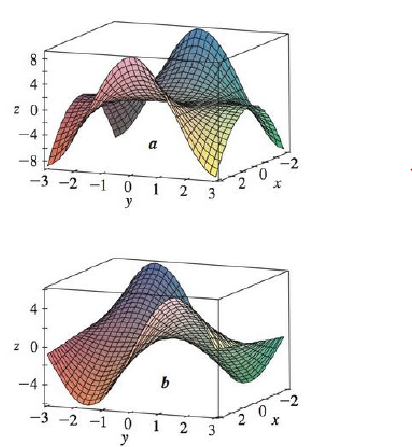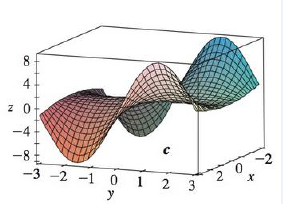Chapter 14.3, Problem 9E

Chapter
Section
Textbook Problem

The following surfaces, labeled a, b, and c, are graphs of a function f and its partial derivatives fx and fy. Identify each surface and give reasons for your choices.To determine

To find: The graphs of a function f and its partial derivatives fx and fy from the given graph of a, b and c.

Explanation

Consider the point (3,3) .

Observe the given graphs and identify that the graph a is increasing and the graphs b and c are decreasing if the point (3,3) move in the positive direction of y.

Hence, the graph of fy is a.

Thus, the remaining two graphs b and c are the graphs of f and fx .

Next look at the point (3,1.5) .

Therefore, it can be concluded that the graph of fx is b and the graph of function f is c

Still sussing out bartleby?

Check out a sample textbook solution.

See a sample solution

The Solution to Your Study Problems

Bartleby provides explanations to thousands of textbook problems written by our experts, many with advanced degrees!

Get Started

Solve the equations in Exercises 126. x+4x+1+x+43x=0

Finite Mathematics and Applied Calculus (MindTap Course List)

Find the derivative of the function. g(x)=12x1+2xtsintdt

Single Variable Calculus: Early Transcendentals, Volume I

In problems 45-62, perform the indicated operations and simplify. 46.

Mathematical Applications for the Management, Life, and Social Sciences